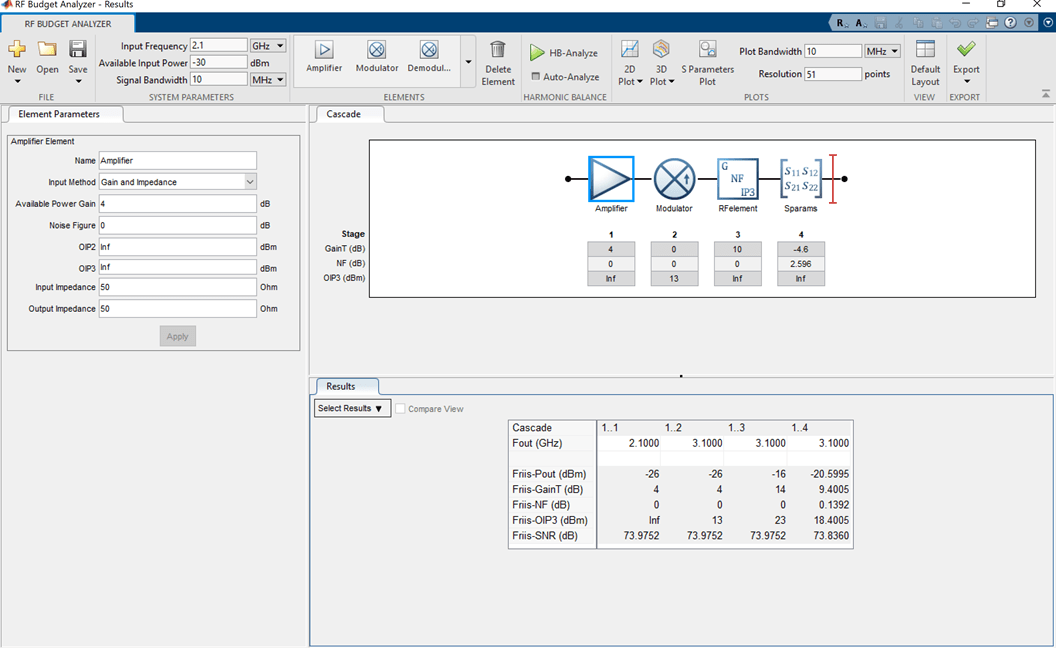Documentation

# modulator

Modulator object

## Description

Use the `modulator` object to create a modulator element. A modulator is a 2-port RF circuit object. You can use this element in the `rfbudget` object and the `circuit` object.

## Creation

### Syntax

``mod = modulator``
``mod = modulator(Name,Value)``

### Description

example

````mod = modulator` creates a modulator object, `mod`, with default property values.```

example

````mod = modulator(Name,Value)` creates a modulator object with additional properties specified by one or more name-value pair arguments. `Name` is the property name and `Value` is the corresponding value. You can specify several name-value pair arguments in any order as `Name1`, `Value1`, `...`, `NameN`, `ValueN`. Properties not specified retain their default values.```

## Properties

expand all

Name of modulator, specified as the comma-separated pair consisting of `'Name'` and a character vector. All names must be valid MATLAB® variable names.

Example: `'Name','mod'`

Available power gain, specified as a nonnegative scalar in dB.

Example: `'Gain',10`

Noise figure, specified as a real finite nonnegative scalar in dB.

Example: `'NF',-10`

Output third-order intercept, specified as a scalar in dBm

Example: `'OIP3',10`

Local oscillator frequency, specified as a real finite positive scalar in Hz.

Example: `'LO',2e9`

Type of modulator, specified as `'Down'` or `'Up'`

Example: `'ConverterType','Up'`

Input impedance, specified as a positive real part finite scalar in ohms. You can also use a complex value with a positive real part.

Example: `'Zin',40`

Output impedance, specified as a scalar in ohms. You can also use a complex value with a positive real part.

Example: `'Zout',40`

Number of ports, specified as a scalar integer. This property is read-only.

Names of port terminals, specified as a cell vector. This property is read-only.

## Examples

collapse all

Create a downconverter modulator with a local oscillator (LO) frequency of 100 MHz.

`m = modulator('ConverterType','Down','LO',100e6)`
```m = modulator: Modulator element Name: 'Modulator' Gain: 0 NF: 0 OIP3: Inf LO: 100000000 ConverterType: 'Down' Zin: 50 Zout: 50 NumPorts: 2 Terminals: {'p1+' 'p2+' 'p1-' 'p2-'} ```

Create a modulator object with a gain of 4 dB and local oscillator (LO) frequency of 2.9 GHz. Create another modulator object that is an upconverter and has an output third-order intercept (OIP3) of 13 dBm.

```mod1 = modulator('Gain',4,'LO',2e9); mod2 = modulator('OIP3',13,'ConverterType','Up');```

Build a 2-port circuit using the modulators.

`c = circuit([mod1 mod2])`
```c = circuit: Circuit element ElementNames: {'Modulator' 'Modulator_1'} Elements: [1x2 modulator] Nodes: [0 1 2 3] Name: 'unnamed' NumPorts: 2 Terminals: {'p1+' 'p2+' 'p1-' 'p2-'} ```

Create an amplifier with a gain of 4 dB.

`a = amplifier('Gain',4);`

Create a modulator with an OIP3 of 13 dBm.

`m = modulator('OIP3',13);`

Create an nport using `passive.s2p`.

`n = nport('passive.s2p');`

Create an rf element with a gain of 10 dB.

`r = rfelement('Gain',10);`

Calculate the rf budget of a series of rf elements at an input frequency of 2.1 GHz, an available input power of -30 dB, and a bandwidth of 10 MHz.

`b = rfbudget([a m r n],2.1e9,-30,10e6)`
```b = rfbudget with properties: Elements: [1x4 rf.internal.rfbudget.Element] InputFrequency: 2.1 GHz AvailableInputPower: -30 dBm SignalBandwidth: 10 MHz AutoUpdate: true Analysis Results OutputFrequency: (GHz) [ 2.1 3.1 3.1 3.1] OutputPower: (dBm) [ -26 -26 -16 -20.6] TransducerGain: (dB) [ 4 4 14 9.4] NF: (dB) [ 0 0 0 0.1392] OIP3: (dBm) [ Inf 13 23 18.4] IIP3: (dBm) [ Inf 9 9 9] SNR: (dB) [73.98 73.98 73.98 73.84] ```

Show the analysis in the RF Budget Analyzer app.

`show(b)`